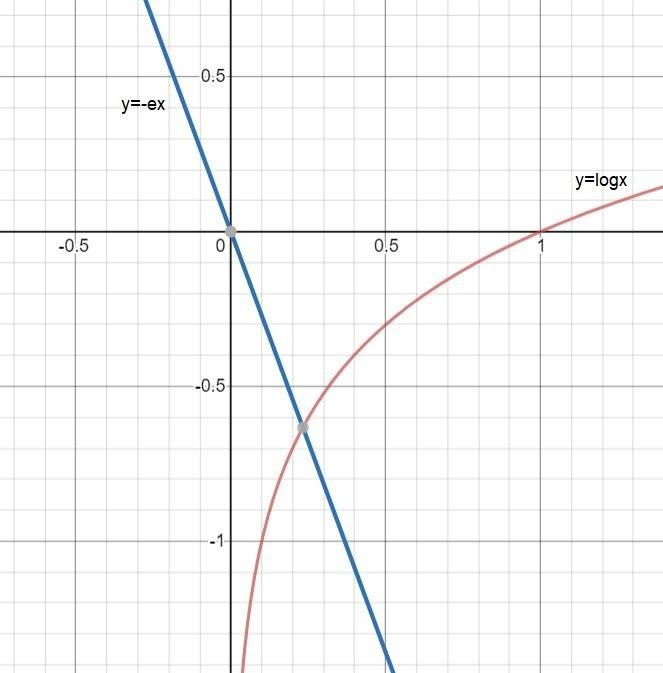QUESTION

# The number of real roots of the equation ${\log _e}x + ex = 0$${\text{A}}{\text{. 0(zero)}} \\ {\text{B}}{\text{. 1}} \\ {\text{C}}{\text{. 2}} \\ {\text{D}}{\text{. 3}} \\$

Hint: -You can solve this question by two method first simply solve and get value of x and check whether it is real or not and we can see there is only two terms so, put one term one side of equal to and another term on opposite side of equal to and draw graph of both to solve further.

Complete step-by-step solution -
From given
${\log _e}x + ex = 0$
You can write it as
${\log _e}x = - ex$
Now you have to draw the graph of $y = {\log _e}x$ and graph of y = -ex .
You should have knowledge of graphs of logarithmic function and also knowledge of graphs of straight line. Now you have to check the cutting points of both the graphs.
So, you can see in diagram graph cuts each other at only one point so there is only one real root of the equation.
Hence option B is the correct option.

Note: -Whenever you get this type of question the key concept of solving is you have to draw a graph of function and check the cutting points. Real roots means simply cutting points of the graphs. But you should have knowledge of drawing graphs. Drawing graphs and checking real roots are the best way of solving a number of real roots questions.